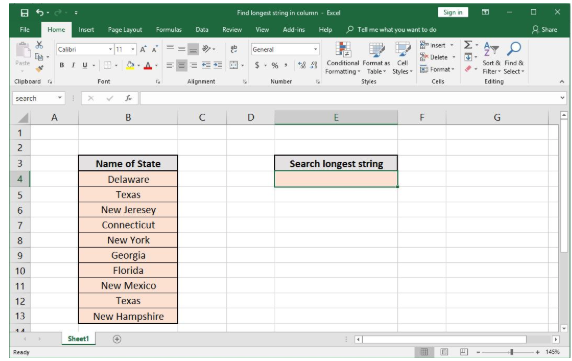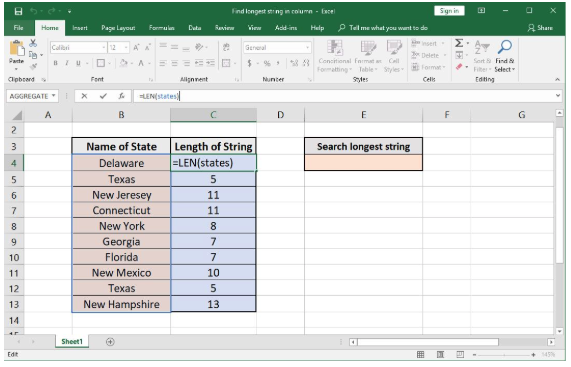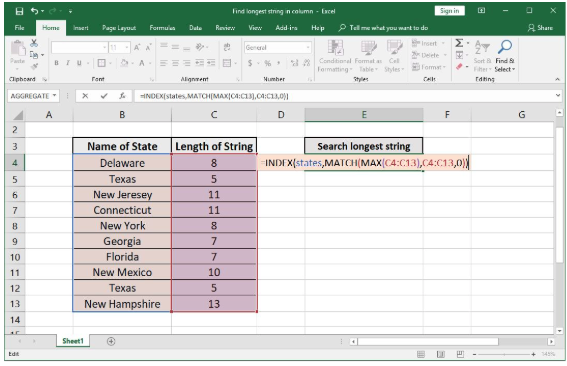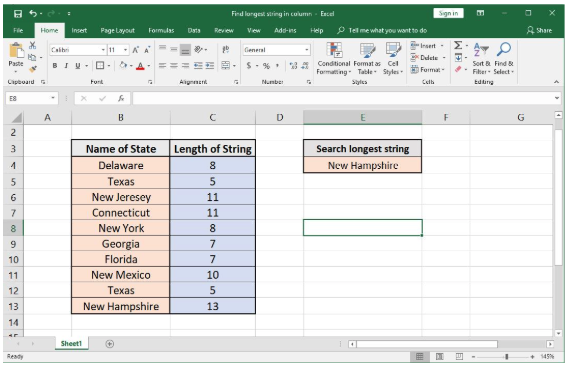Get instant live expert help with Excel or Google Sheets“My Excelchat expert helped me in less than 20 minutes, saving me what would have been 5 hours of work!”

#### Post your problem and you’ll get Expert help in seconds.

Your message must be at least 40 characters
Our professional Expert are available now. Your privacy is guaranteed.

# How to Find the Longest String in a Column

If there is an array of string and there is a need to find the longest string that has the maximum number of characters in it as compared to other entries. The formula discussed in this article can be used to do this in a simpler way.

## Find the Longest String in a Column

### Formula using several functions

`=INDEX(data,MATCH(MAX(LEN(data)),LEN(data),0))`

### Explanation of formula

The above written generic formula makes use of Length (LEN), Maximum (MAX), MATCH and INDEX functions. A brief review of these functions and the working of the formula is discussed below.

### How does the Formula to Find Longest String in Column Work?

First, the formula to find the longest string in a column works with the coordination of 4 formulas. The Length (LEN) function is used to return the length of each string in an array. For example, the length of the word “Old” is 3, as it contains 3 characters.

Second, the MAX function is used to return the maximum value from an array provided to it as an argument. The MAX function, with the help of the LEN function, returns the number of characters of an entry in the array with the maximum length.

Third, to get the position of the value with the maximum number of characters from the array, the MATCH function is used. This function is configured to get the exact match by providing the 3rd parameter as 0 for this purpose. This function returns the position of that entry in the array which is passed to INDEX function as the row argument.

This INDEX function looks for that entry in the array and returns the string contained in that specific cell.

### Example

A list of states of U.S. has been considered in the form of a list in column B of Excel. To find the longest string from it, the following sheet has been considered as an example.Figure1. Sample sheet to find the longest string in column

To find the longest string, first, find the length of each string in the array named as “states” ranging from B4 to B13. Enter the following formula in cells C4:C13.

`=LEN(states)`Figure2. Finding the length of each string in the column

Now, enter the following formula in E4 to get the longest string in the column as a result:

`=INDEX(states,MATCH(MAX(C4:C13),C4:C13,0))`Figure3. Entering the formula in E4 to find the longest string from array “states”, using C4:C13 as the array for Length of Strings

The result of the above-entered command can be seen in the figure below:Figure4. The result of finding the longest string in column

It can be seen that the maximum number of string length in column C4:C13 is 13, which corresponds to New Hampshire.

Still need some help with Excel formatting or have other questions about Excel? Connect with a live Excel expert here for some 1 on 1 help. Your first session is always free.

### Did this post not answer your question? Get a solution from connecting with the expert.Another blog reader asked this question today on Excelchat:
Solution examplesindex and match with duplicates. I need to use another column as a reference, so my return value has two match the value of two things for it to return
Solved by E. H. in 60 minsI need a formula to calculate the MAX of a range of 167 rows in column D, then find the MAX of the next 167 rows in column D and so on continuously through the entire sheet. Example MAX D2:D169, D170:D337, D338:D505 etc...
Solved by I. Q. in 60 minsI need a formula that will compare multiple columns, and return the column header name of whichever column had the highest value. I am using (INDEX(\$F\$1:\$K\$1,0,MATCH(MAX(F2:K2),F2:K2,0))) , but one row had all the same values and the formula returned the first column header as being the "winner". This formula cannot distinguish when there are ties.
Solved by M. C. in 51 minsHello, I have a big spreadsheet in which I need to know how many patients came in each month based on provider. I am using the following formula but I still get the N/A error. =INDEX('No Show Appts Data'!D:E, MATCH(1, 'No Show Appts Data'!D:D='No Shows Data'!M2)*('No Shows Data'!E:E='No Shows Data'!N1),0)) No Show Appts Data is the name of the sheet where Column D is Month Year of date in question and Column E is the provider. No Shows Data is the sheet where I am making all the formula calculation where Column M is Month and Year and Column N is the provider in question and therefore M2 is the month in question and N1 the provider in question. How do I fix this error? Thanks
Solved by F. H. in 40 minsI need to find an INDEX function that will convert the Call Day (a number 1-7) to the actual weekday found in row 1 of the DayofWeek named range. I don't know if I'm supposed to use the MATCH function as well or not.
Solved by D. D. in 11 mins## Subscribe to Excelchat.coAnother blog reader asked this question today on Excelchat: